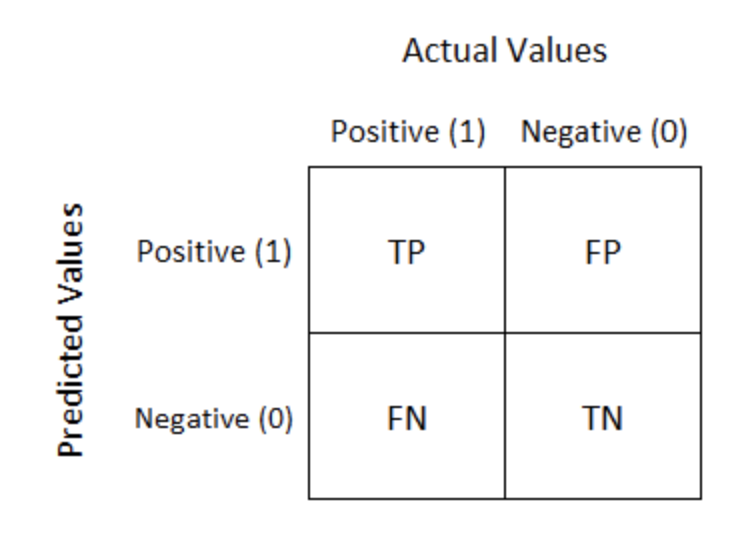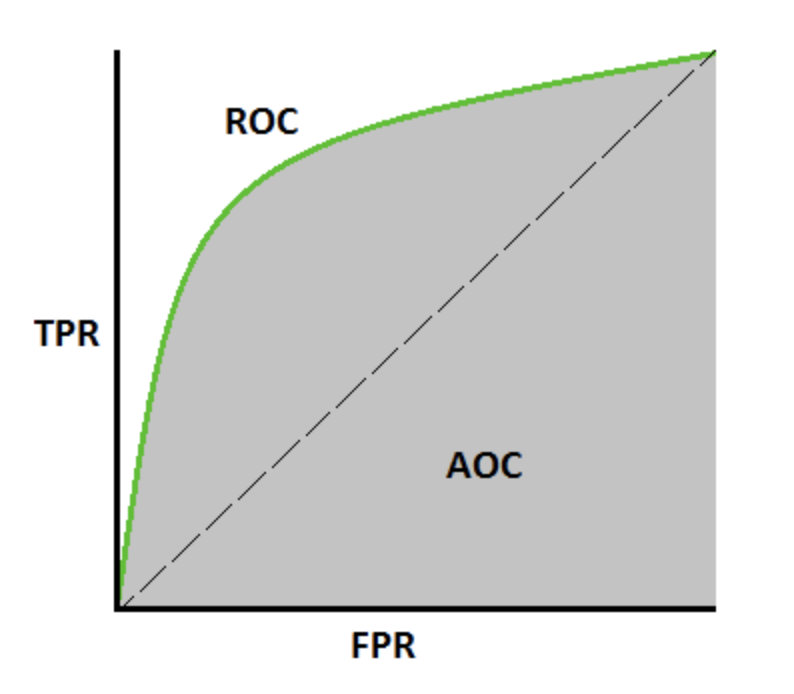## Basics for binary dependent variables.

Suppose you are interested in forecasting the onset of a binary variable, e.g. attack/no attack. You run a logistic regression model (logit) and calculate predicted values for the next time step. Let’s call these predictions $$\hat y$$. $$\hat y$$ will take on values anywhere between 0 and 1. The true values, $$y$$, are either 1s or 0s. So practically you might end up with something like that:

yhat <- runif(0,1, n = 10)
y <- sample(c(0,1), size = 10, replace=T)
this.data1 <- data.frame(yhat, y)
this.data1
##          yhat y
## 1  0.01547187 1
## 2  0.57286281 0
## 3  0.53792174 1
## 4  0.34291858 0
## 5  0.98467503 0
## 6  0.70840483 0
## 7  0.47307656 0
## 8  0.82070093 1
## 9  0.64889212 1
## 10 0.65151702 1

Now we want to decide how good' these predictions are. One simple way is to choose a threshold, say 0.5. Any prediction above 0.5 (i.e., $\hat y > 0.5$) is then classified as an attack (i.e., 1), and anything below is classified asno attack’. Thus:

yhat <- runif(0,1, n = 10)

yhatThreshold <- rep('negative', 10)
yhatThreshold[yhat > 0.5] <- 'positive'

y <- sample(c(0,1), size = 10, replace=T)
y[y==0] <- 'negative'
y[y==1] <- 'positive'
this.data <- data.frame(yhatThreshold, y)
this.data
##    yhatThreshold        y
## 1       negative positive
## 2       positive negative
## 3       negative positive
## 4       negative negative
## 5       positive positive
## 6       positive positive
## 7       negative negative
## 8       positive positive
## 9       positive positive
## 10      negative negative

Now we can write this in a confusion matrix. A confusion matrix is a matrix with 4 possible combinations of predicted and actual valuesSo let’s try this:

library(caret)
## Loading required package: lattice
## Loading required package: ggplot2
library(e1071)
confusionMatrix( factor(this.data$yhatThreshold), factor(this.data$y))
## Confusion Matrix and Statistics
##
##           Reference
## Prediction negative positive
##   negative        3        2
##   positive        1        4
##
##                Accuracy : 0.7
##                  95% CI : (0.3475, 0.9333)
##     No Information Rate : 0.6
##     P-Value [Acc > NIR] : 0.3823
##
##                   Kappa : 0.4
##
##  Mcnemar's Test P-Value : 1.0000
##
##             Sensitivity : 0.7500
##             Specificity : 0.6667
##          Pos Pred Value : 0.6000
##          Neg Pred Value : 0.8000
##              Prevalence : 0.4000
##          Detection Rate : 0.3000
##    Detection Prevalence : 0.5000
##       Balanced Accuracy : 0.7083
##
##        'Positive' Class : negative
## 

First it gives us a nice table. In it, we can see the - True positive (TP): correct positive prediction - False positive (FP): incorrect positive prediction - True negative (TN): correct negative prediction - False negative (FN): incorrect negative prediction

But it also gives us important metrics.

One is called accuracy and is calculated as $ACC = \frac{(TP+TN)}{P+N}$. The other is the error rate and is just the flipside of accuracy: $ERR = \frac{FP+FN}{P+N}$. Note that $$ERR = 1-ACC$$.

Accuracy answers the following question: Out of all the cases we’re looking at, how many did we correctly label (i.e., how many TP and TN out of all cases)?

Note: Accuracy is a good measure if your data is balanced (i.e., equal number of 1s and 0s). If not, then you can easily get a high accuracy by predicting all your results as one class (e.g., all 0s)

Precision is the ratio of cases we’ve correctly labeled as positive to all those we’ve labeled as positive. $Precision = \frac{TP}{TP+FP}$ Why does this matter? If precision is high, it means that whenever we announce an event, it tends to happen.

Recall answers a slightly different question: of all the positive cases, how many did we correctly predict? $Recall = \frac{TP}{TP+FN}$ This differs from precision. We could have perfect precision by predicting one single positive event (out of a thousand) and that event turns out to happen. But we’ve missed the 999 other events that did happen (we predicted them as negatives). Recall punishes this kind of errors: Recall decreases as FN increase. On the other hand, we can have a high recall if we predit everything as 1s. Then TP=1000 and FN=0, so recall = 1!

As you can see, there is an important trade-off there between Precision and Recall. It is easy to do well on one of them, but hard to do well on both. That’s why it is important to report both.

Specificity is the ratio of the number of correctly labeled negatives to the number of negatives in reality. $Specificity = \frac{TN}{TN+FP}$

The problem with the confusion matrix is that it only works for a given threshold. E.g. here, we have set the threshold at 0.5. Anything higher was classified as 1, anything lower as 0. But what happens if we set the threshold to 0.4? to 0.1? to 0.9? Would that changes our results? Yes, and we’d like to know how. One way to represent this is with curves that show some of the metrics above for all possible thresholds.

The ROC curve represents the trade-off between False positive rate (less is better) and true positive rate (more is better). It is easy to get a TPR by just calling every observation as 1. But that also means having lots of false positives. Conversely, we can get few FP by just calling everything a 0. But that implies a low number of TP. This trade-off is represented by the ROC curve.In an ideal world all the observations we classify as less than our threshold would be true 0s and the ones we classify as greater than our threshold would be true 1s: